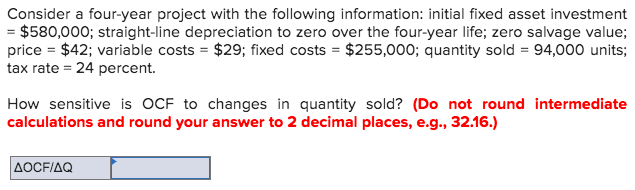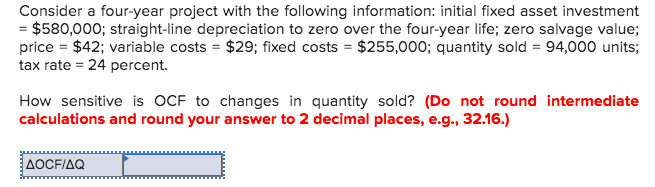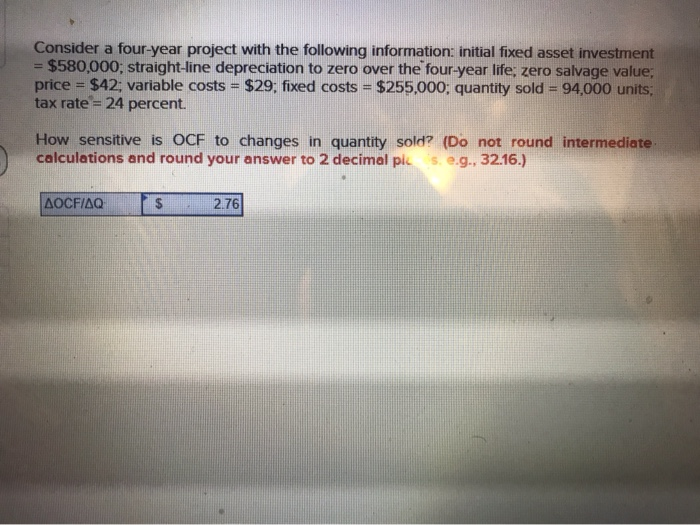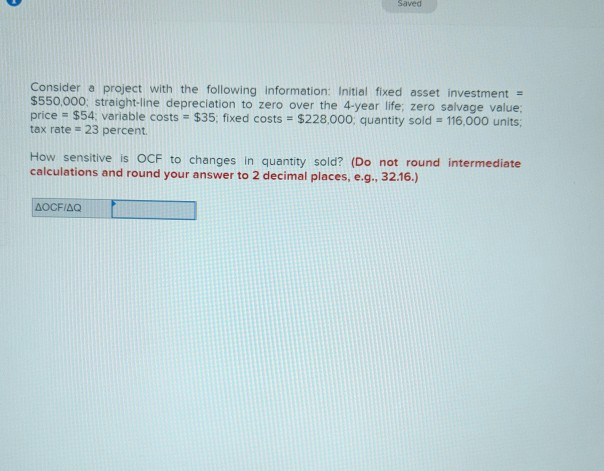# Consider a four-year project with the following information: initial fixed asset investment = \$474797; straight-line depreciation...

Consider a four-year project with the following information: initial fixed asset investment = \$474797; straight-line depreciation to zero over the four-year life; zero salvage value; price = \$32; variable costs = \$22; fixed costs = \$193539; quantity sold = 78542 units; tax rate = 34 percent. Calculate the sensitivity of the OCF to changes in the quantity sold.

[Hint: Think of this as, "How much does OCF change if we can sell one more unit each year?"]

(Do not round intermediate calculations and round your final answer to 2 decimal places. Omit the "\$" sign and commas in your response. For example, \$1.2345 should be entered as 1.23.)

This Homework Help Question: "Consider a four-year project with the following information: initial fixed asset investment = \$474797; straight-line depreciation..." No answers yet.

We need 10 more requests to produce the answer to this homework help question. Share with your friends to get the answer faster!

0 /10 have requested the answer to this homework help question.

Once 10 people have made a request, the answer to this question will be available in 1-2 days.
All students who have requested the answer will be notified once they are available.
##### Add Answer to: Consider a four-year project with the following information: initial fixed asset investment = \$474797; straight-line depreciation...
Similar Homework Help Questions
• ### Consider a four-year project with the following information: initial fixed asset investment = \$275,000; straight-line depreciation...

Consider a four-year project with the following information: initial fixed asset investment = \$275,000; straight-line depreciation to zero over the four-year life; zero salvage value; price = \$46; variable costs = \$12; fixed costs = \$195,000; quantity sold = 84,000 units; tax rate = 34%. How sensitive is OCF to changes in quantity sold? (Do not round intermediate calculations. Round the final answer to 2 decimal places. Omit \$ sign in your response.) (Do not forget to include + or...

• ### Consider a four-year project with the following information: initial fixed asset investment = \$575,000; straight-line depreciation...

Consider a four-year project with the following information: initial fixed asset investment = \$575,000; straight-line depreciation to zero over the four-year life; zero salvage value; price = \$36; variable costs = \$17; fixed costs = \$175,000; quantity sold = 84,000 units; tax rate = 34%. How sensitive is OCF to changes in quantity sold? (Do not round intermediate calculations. Round the final answer to 2 decimal places. Omit \$ sign in your response.) (Do not forget to include + or...

• ### Consider a four-year project with the following information: initial fixed asset investment = \$375,000; straight-line depreciation...

Consider a four-year project with the following information: initial fixed asset investment = \$375,000; straight-line depreciation to zero over the four-year life; zero salvage value; price = \$56; variable costs = \$23; fixed costs = \$195,000; quantity sold = 84,000 units; tax rate = 34%. How sensitive is OCF to changes in quantity sold? (Do not round intermediate calculations. Round the final answer to 2 decimal places. Omit \$ sign in your response.) (Do not forget to include + or...

• ### Consider a four-year project with the following information: initial fixed asset investment = \$375,000; straight-line depreciation...

Consider a four-year project with the following information: initial fixed asset investment = \$375,000; straight-line depreciation to zero over the four-year life; zero salvage value; price = \$56; variable costs = \$23; fixed costs = \$195,000; quantity sold = 84,000 units; tax rate = 34%. How sensitive is OCF to changes in quantity sold? (Do not round intermediate calculations. Round the final answer to 2 decimal places. Omit \$ sign in your response.) (Do not forget to include + or...

• ### Question#2 - Consider a four-year project with the following information: initial fixed asset investment = \$575,000;...

Question#2 - Consider a four-year project with the following information: initial fixed asset investment = \$575,000; straight-line depreciation to zero over the four-year life; zero salvage value; price = \$36; variable costs = \$17; fixed costs = \$175,000; quantity sold = 84,000 units; tax rate = 34%. How sensitive is OCF to changes in quantity sold? (Do not round intermediate calculations. Round the final answer to 2 decimal places. Omit \$ sign in your response.) (Do not forget to include...

• ### Consider a four-year project with the following information: initial fixed asset investment = \$570,000; straight-line depreciation...

Consider a four-year project with the following information: initial fixed asset investment = \$570,000; straight-line depreciation to zero over the four-year life; zero salvage value; price = \$40; variable costs = \$27; fixed costs = \$245,000; quantity sold = 88,000 units; tax rate = 22 percent.    How sensitive is OCF to changes in quantity sold? (Do not round intermediate calculations and round your answer to 2 decimal places, e.g., 32.16.)

• ### Consider a four-year project with the following information: initial fixed asset investment = \$580,000; straight-line depreciation...Consider a four-year project with the following information: initial fixed asset investment = \$580,000; straight-line depreciation to zero over the four-year life; zero salvage value; price = \$42; variable costs = \$29; fixed costs = \$255,000; quantity sold = 94,000 units; tax rate = 24 percent. How sensitive is OCF to changes in quantity sold? (Do not round intermediate calculations and round your answer to 2 decimal places, e.g., 32.16.) AOCFIAQ

• ### Consider a four-year project with the following information: initial fixed asset investment = \$580,000; straight-line depreciation...Consider a four-year project with the following information: initial fixed asset investment = \$580,000; straight-line depreciation to zero over the four-year life; zero salvage value; price = \$42; variable costs = \$29; fixed costs = \$255,000; quantity sold = 94,000 units; tax rate = 24 percent. How sensitive is OCF to changes in quantity sold? (Do not round intermediate calculations and round your answer to 2 decimal places, e.g., 32.16.) AOCFAQſ

• ### Consider a four-year project with the following information: initial fixed asset investment = \$580,000; straight-li...Consider a four-year project with the following information: initial fixed asset investment = \$580,000; straight-line depreciation to zero over the four-year life; zero salvage value, price = \$42; variable costs = \$29; fixed costs = \$255,000; quantity sold = 94.000 units; tax rate = 24 percent. How sensitive is OCF to changes in quantity sold? (Do not round intermediate calculations and round your answer to 2 decimal pis. e.g., 32.16.) LOCFIAQ S 2.76

• ### Consider a project with the following information: Initial fixed asset investment - \$550,000 straight-line depreciation to...Consider a project with the following information: Initial fixed asset investment - \$550,000 straight-line depreciation to zero over the 4-year life; zero salvage value: ole costs = \$35: fixed costs = \$228,000, quantity sold = 116,000 units tax rate = 23 percent. How sensitive is OCF to changes in quantity sold? (Do not round intermediate calculations and round your answer to 2 decimal places, e.g., 32.16.) AOCFIAQ

Free Homework App